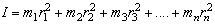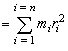# Physics Homework Help With Moment of Inertia

## Moment of Inertia

One of the most fundamental characteristics possessed by an object is its intrinsic reluctance to accept a change in its state of motion i.e. its inertia

A body needs a force to start its translational inertial is better know as mass.

The measure of a body's rotational inertia is called moment of inertia and it is represented by I. The moment of inertia of a body is a function of the mass of the body, the distribution of that mass and the position of the axis of rotation.

Consider a particle of mass m situated at a distance r from the axis as shown in figure. Its motion of inertia I is defined as

I = mr2 … (v)If a system of particles is made of number of particles of masses m1, m2, m3 …. mn at distance r1, r2, r3, …. rn from the axis of rotation, its moment of inertia is defined as… (vi)

### Homework Help For Moment of Inertia

assignmenthelp.net provides best Online Assignment Help service in Physics for all standards. Our Tutor provide their high quality and optimized Tutorial help to fulfill all kind of need of Students.